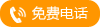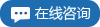•YD系列隔膜式液 `` ``
•无线压力变送器 `` ``
•高防护型温度变 `` ``
•分体式电磁流 `` ``
•高防护型温度变 `` ``
•电动真空源 `` ``
•温压补偿一体化 `` ``
•雷达式水位计 `` ``
•双法兰液位变送 `` ``
•YD系列单晶硅智 `` ``
•液晶显示金属管 `` ``
•台式液体压力源 `` ``
•电容式液位计 `` ``
•YTZ型电阻远传压 `` ``
•液体涡轮流量计 `` ``
•双法兰液位变送 `` ``
•液晶显示金属管 `` ``
•YD系列单晶硅智 `` ``
•超声波液位计 `` ``
•隔膜式双法兰液 `` ``